Thermal Stress of a Cylinder using Axisymmetric Elements

A steel cylinder with inner radius 5 inches and outer radius 10 inches is 40 inches long and has spherical end caps.  The interior of the cylinder is kept at 75 deg F, and heat is lost on the exterior by convection to a fluid whose temperature is 40 deg F.   The convection coefficient is 0.56 BTU/hr-sq.in-F.  Calculate the stresses in the cylinder caused by the temperature distribution.

The problem is solved in two steps. First, the geometry is created, the preference set to 'thermal', and the heat transfer problem is modeled and solved.  The results of the heat transfer analysis are saved in a file 'jobname.RTH' (Results THermal analysis) when you issue a save jobname.db command.

Next the heat transfer boundary conditions and loads are removed from the mesh, the preference is changed to 'structural', the element type is changed from 'thermal' to 'structural', and the temperatures saved in 'jobname.RTH' are recalled and applied as loads.

1. Start ANSYS and assign a job name to the project.  Run Interactive -> set working directory and jobname.

2.  Preferences -> Thermal will show -> OK

3.  A quadrant of a section through the cylinder is created using ANSYS area creation tools.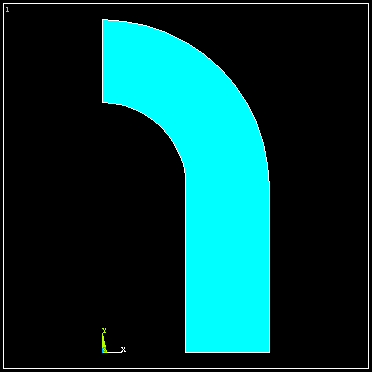4.  Preprocessor -> Element Type -> Add/Edit/Delete -> Add -> Solid 8 node 77 -> OK ->

Options -> K3 Axisymmetric -> OK

5.  Preprocessor -> Material Props -> Isotropic -> Material Number 1 -> OK

EX = 3.E7 (psi)

DENS = 7.36E-4 (lb sec^2/in^4)

ALPHAX = 6.5E-6

PRXY = 0.3

KXX = 0.69 (BTU/hr-in-F)

6. Mesh the area using methods discussed in previous examples.

7.  Preprocessor -> Loads -> Apply -> Temperatures -> Nodes

Select the nodes on the interior and set the temperature to 75.

8.   Preprocessor -> Loads -> Apply -> Convection -> Lines

Select the lines defining the outer surface and set the coefficient to 0.56 and the fluid temp to 40.

9.  Preprocessor -> Loads -> Apply -> Heat Flux -> Lines

Select the vertical and horizontal lines of symmetry and set the heat flux to zero.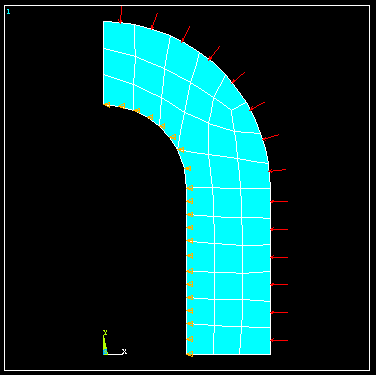10.  Solution -> Solve current LS

11. General Postprocessor -> Plot Results -> Nodal Solution  -> Temperature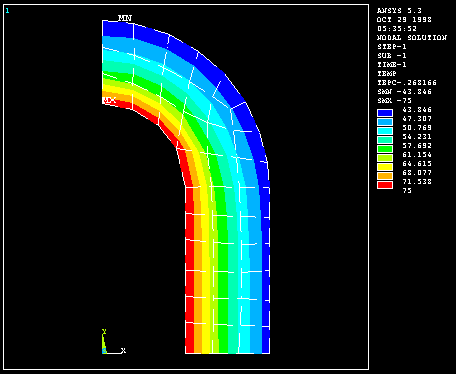The temperature on the interior is 75 F and on the outside wall it is found to be 43.

12.  File -> Save Jobname.db

13.  Preprocessor -> Loads -> Delete -> Delete All -> Delete All Opts.

14.  Preferences -> Structural will show, Thermal will NOT show.

15.  Preprocessor -> Element Type -> Switch Element Type -> OK (This changes the element to structural)

16.  Preprocessor -> Loads -> Apply -> Displacements -> Nodes

(Fix nodes on vertical and horizontal lines of symmetry from crossing the lines of symmetry.)

17.  Preprocessor -> Loads -> Apply -> Temperature -> From Thermal Analysis

Select Jobname.RTH (If it isn't present, look for the default 'file.RTH' in the root directory)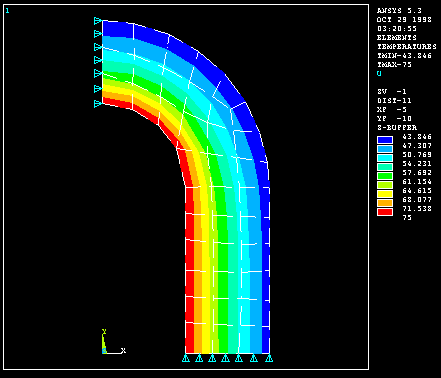18.  Solution -> Solve Current LS

19.  General Postprocessor -> Plot Results -> Element Solution - von Mises Stress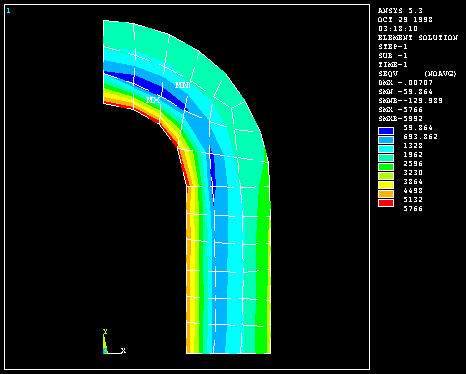The von Mises stress is seen to be a maximum in the end cap on the interior of the cylinder and would govern a yield-based design decision.

Back